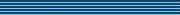### Maximal exponents of polyhedral cones

Speaker
Prof. R. Loewy
Duration
48:32
Date
17 October 2011
Abstract
Let K be a proper (i.e., closed, pointed, full and convex) cone in R^n. We consider A∈R^(n×n) which is K-primitive, that is, there exists a positive integer l such that A^l.x ∈ int K for every 0≠x∈K. The smallest such l is called the exponent of A, denoted by γ(A).

For a polyhedral cone K, the maximum value of γ(A), taken over all K-primitive matrices A, is denoted by γ(K). Our main result is that for any positive integers m,n, 3 ≤ n ≤ m, the maximum value of γ(K), as K runs through all n-dimensional polyhedral cones with m extreme rays, equals

( n - 1 )( m - 1 ) + ½( 1 + (-1)^{(n-1)m} ).

We will consider various uniqueness issues related to the main result as well as its connections to known results.

This talk is based on a joint work with Micha Perles and Bit-Shun Tam.
You should see the video here…
High quality
Fullscreen
Volume